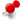# Where does my code spend time and how to improve thisClassicListThreaded5 messagesOpen this post in threaded view
|

## Where does my code spend time and how to improve this

 I have a simple kernel (see below)  that basically does tool_size**2 b[] = min(a[],b[]) ops between two float arrays (height maps). When I queue 100.000 ops of this kernel for tool_size = 8 I get about 100.000 completed ops/sec and for tool_size =128 I get about 50.000 So my question is, given that even if I reduce my kernel to almost nil I get similar results, what is the limiting factor here and what I can do about it. wbr Kusti for (int k = 0; k < 100000; k++) {         kernel.setArg(2, tool_pos_x);         kernel.setArg(3, tool_pos_y);         kernel.setArg(4,tool_pos_z);         queue.put2DRangeKernel(kernel, 0, 0, tool_size, tool_size, 0, 0);//         } kernel void millcut(         global const float* tool,   global float* stock,   int tool_pos_x,   int tool_pos_y,   int tool_pos_z   ) {   int x=get_global_id(0);   int y=get_global_id(1);   int si = (x + tool_pos_x) + (y + tool_pos_y) * stock_size;         int ti = x + y * tool_size;         int h=tool[ti]+tool_pos_z;         if (stock[si] > h)                 stock[si] = h; }
Open this post in threaded view
|

## Re: Where does my code spend time and how to improve this

 Administrator Hard to be certain, but for higher performance I'd advise putting more of the work into the kernel instead of the host code. If you're looping over tool_pos_x|y|z for example, having that loop inside the kernel might give better performance. Another possibility is that since your kernel code has a data-dependent branch (if (stock[si] > h)), it may not parallelize onto a GPU very well. For GPU efficiency, a whole "warp" of threads needs to branch the same direction most of the time -- the more divergence there is within the warp, the lower the performance. Yet another possibility is that you're doing integer math here instead of floating-point, which many GPUs are poor at. Your types are also a little strange -- you're reading a float from "tool", but then converting to int before you write to "stock". You should clearly separate index calculations (int) from data copying (float).
Open this post in threaded view
|

## Re: Where does my code spend time and how to improve this

 Thanks for taking a look. For test I simplified the kernel to: int x=get_global_id(0); int y=get_global_id(1); int si = (x + tool_pos_x) + (y + tool_pos_y) * stock_size; stock[si] = 0.0f; And it makes no difference. So the limiting factor must be the kernel scheduling time? For that of course doing more work inside the kernel would work, I will try that. Yes, you are right about the float/int mix. Basically everything is int so I will fix that. The data dependent stuff needs to be there, it is the beef of the calculation. As far as I understand (which is not much at this point) the execution time will be the sum of code path execution times in and since there is no else part in the if I think this should just execute at the speed as if every statement is executed. The integer math not being GPU strong side, you are of course right. On the other hand there is only integer addition and comparison ahd the multiplications are variable by constants and are the sort of index calculations that every kernel needs to do so I expect OpenCL/GPU must be pretty efficient at those. And my test above would seem to indicate that indeed the actual work done inside kernel does not matter much. So I guess I will have to go and try get more work done in the kernel. Thanks again! wbr Kusti